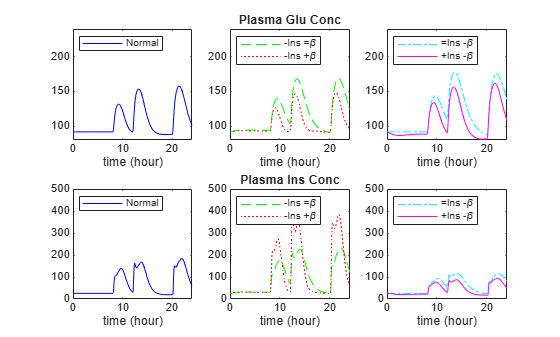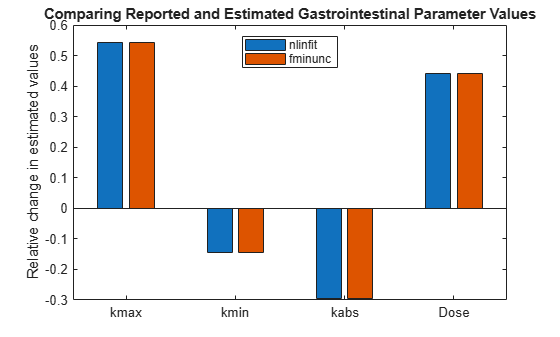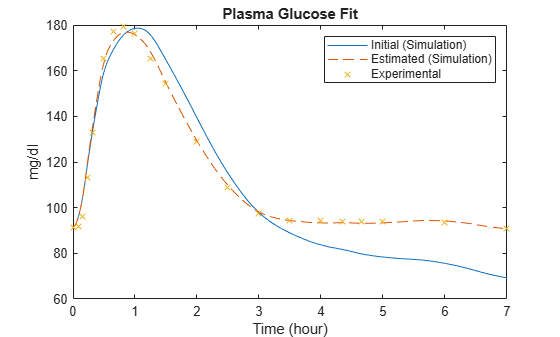Documentation

This is machine translation

Mouseover text to see original. Click the button below to return to the English version of the page.

Simulating the Glucose-Insulin Response

This example shows how to simulate and analyze a model in SimBiology® using a physiologically based model of the glucose-insulin system in normal and diabetic humans.

This example requires Statistics and Machine Learning Toolbox™ and Optimization Toolbox™.

References

1. Meal Simulation Model of the Glucose-Insulin System. C. Dalla Man, R.A. Rizza, and C. Cobelli. IEEE Transactions on Biomedical Engineering (2007) 54(10), 1740-1749.

2. A System Model of Oral Glucose Absorption: Validation on Gold Standard Data. C. Dalla Man, M. Camilleri, and C. Cobelli. IEEE Transactions on Biomedical Engineering (2006) 53(12), 2472-2478.

Aims

• Implement a SimBiology model of the glucose-insulin response.

• Simulate the glucose-insulin response to one or more meals for normal and impaired (diabetic) subjects.

• Perform parameter estimation using sbiofit with a forcing function strategy.

Background

In their 2007 publication, Dalla Man et al. develop a model for the human glucose-insulin response after a meal. This model describes the dynamics of the system using ordinary differential equations. The authors used their model to simulate the glucose-insulin response after one or more meals, for normal human subjects and for human subjects with various kinds of insulin impairments. The impairments were represented as alternate sets of parameter values and initial conditions.

We implemented the SimBiology model, m1, by:

• Translating the model equations in Dalla Man et al. (2007) into reactions, rules, and events.

• Organizing the model into two compartments, one for glucose-related species and reactions (named Glucose appearance) and one for insulin-related species and reactions (named Insulin secretion).

• Using the parameter values and initial conditions from the model equations and from Table 1 and Figure 1.

• Including an equation for the gastric emptying rate as presented in Dalla Man et al. (2006).

• Setting the units for all species, compartments, and parameters as specified by Dalla Man et al. (2007), which allows the SimBiology model to be simulated using unit conversion. (Note that SimBiology also supports the use of dimensionless parameters by setting their ValueUnits property to dimensionless.)

• Setting the configuration set TimeUnits to hour, since simulations were conducted over 7 or 24 hours.

• Using a basis of 1 kilogram of body weight to transform species and parameters that were normalized by body weight in the original model. Doing so made species units in amount or concentration, as required by SimBiology.

We represented the insulin impairments in the SimBiology model as variant objects with the following names:

• Type 2 diabetic

• Low insulin sensitivity

• High beta cell responsivity

• Low beta cell responsivity

• High insulin sensitivity

We represented the meals in the SimBiology model as dose objects:

• A dose named Single Meal represents a single meal of 78 grams of glucose at the start of a simulation.

• A dose named Daily Life represents one day's worth of meals, relative to a simulation starting at midnight: breakfast is 45 grams of glucose at 8 hours of simulation time (8 a.m.), lunch is 70 grams of glucose at 12 hours (noon), and dinner is 70 grams of glucose at 20 hours (8 p.m.).

A diagram of the SimBiology model is shown below:Setup

sbioloadproject('insulindemo', 'm1')

Suppress an informational warning that is issued during simulations.

warnSettings = warning('off', 'SimBiology:DimAnalysisNotDone_MatlabFcn_Dimensionless');

Simulating the Glucose-Insulin Response for a Normal Subject

Select the Single Meal dose object and display its properties.

mealDose = sbioselect(m1, 'Name', 'Single Meal'); get(mealDose)
 Active: 0 Annotation: '' Name: 'Single Meal' Notes: '' Parent: [1x1 SimBiology.Model] Tag: '' Type: 'repeatdose' UserData: [] DurationParameterName: '' LagParameterName: '' TargetName: 'Dose' EventMode: 'stop' AmountUnits: 'gram' RateUnits: '' TimeUnits: 'hour' Amount: 78 Interval: 0 Rate: 0 RepeatCount: 0 StartTime: 0 

Simulate for 7 hours.

configset = getconfigset(m1,'active'); configset.StopTime = 7;

Display the simulation time units (and StopTime units).

configset.TimeUnits
ans = 'hour' 

Simulate a single meal for a normal subject.

normalMealSim = sbiosimulate(m1, configset, [], mealDose);

Simulating the Glucose-Insulin Response for a Type 2 Diabetic

Select the Type 2 diabetic variant and display its properties.

diabeticVar = sbioselect(m1, 'Name', 'Type 2 diabetic')
 SimBiology Variant - Type 2 diabetic (inactive) ContentIndex: Type: Name: Property: Value: 1 parameter Plasma Volume ... Value 1.49 2 parameter k1 Value 0.042 3 parameter k2 Value 0.071 4 parameter Plasma Volume ... Value 0.04 5 parameter m1 Value 0.379 6 parameter m2 Value 0.673 7 parameter m4 Value 0.269 8 parameter m5 Value 0.0526 9 parameter m6 Value 0.8118 10 parameter Hepatic Extrac... Value 0.6 11 parameter kmax Value 0.0465 12 parameter kmin Value 0.0076 13 parameter kabs Value 0.023 14 parameter kgri Value 0.0465 15 parameter f Value 0.9 16 parameter a Value 6e-05 17 parameter b Value 0.68 18 parameter c Value 0.00023 19 parameter d Value 0.09 20 parameter Stomach Glu Af... Value 125 21 parameter kp1 Value 3.09 22 parameter kp2 Value 0.0007 23 parameter kp3 Value 0.005 24 parameter kp4 Value 0.0786 25 parameter ki Value 0.0066 26 parameter [Ins Ind Glu U... Value 1 27 parameter Vm0 Value 4.65 28 parameter Vmx Value 0.034 29 parameter Km Value 466.21 30 parameter p2U Value 0.084 31 parameter K Value 0.99 32 parameter alpha Value 0.013 33 parameter beta Value 0.05 34 parameter gamma Value 0.5 35 parameter ke1 Value 0.0007 36 parameter ke2 Value 269 37 parameter Basal Plasma G... Value 164.18 38 parameter Basal Plasma I... Value 54.81 

Simulate a single meal for a Type 2 diabetic.

diabeticMealSim = sbiosimulate(m1, configset, diabeticVar, mealDose);

Compare the results for the most important outputs of the simulation.

• Plasma Glucose (species Plasma Glu Conc)

• Plasma Insulin (species Plasma Ins Conc)

• Endogenous Glucose Production (parameter Glu Prod)

• Glucose Rate of Appearance (parameter Glu Appear Rate)

• Glucose Utilization (parameter Glu Util)

• Insulin Secretion (parameter Ins Secr)

outputNames = {'Plasma Glu Conc', 'Plasma Ins Conc', 'Glu Prod', ... 'Glu Appear Rate', 'Glu Util', 'Ins Secr'}; figure; for i = 1:numel(outputNames) subplot(2, 3, i); [tNormal, yNormal ] = normalMealSim.selectbyname(outputNames{i}); [tDiabetic, yDiabetic] = diabeticMealSim.selectbyname(outputNames{i}); plot( tNormal , yNormal , '-' , ... tDiabetic , yDiabetic , '--' ); % Annotate figures outputParam = sbioselect(m1, 'Name', outputNames{i}); title(outputNames{i}); xlabel('time (hour)'); if strcmp(outputParam.Type, 'parameter') ylabel(outputParam.ValueUnits); else ylabel(outputParam.InitialAmountUnits); end xlim([0 7]); % Add legend if i == 3 legend({'Normal', 'Diabetic'}, 'Location', 'Best'); end endNote the much higher concentrations of glucose and insulin in the plasma, as well as the prolonged duration of glucose utilization and insulin secretion.

Simulating One Day with Three Meals for a Normal Subject

Set the simulation StopTime to 24 hours.

configset.StopTime = 24;

Select daily meal dose.

dayDose = sbioselect(m1, 'Name', 'Daily Life');

Simulate three meals for a normal subject.

normalDaySim = sbiosimulate(m1, configset, [], dayDose);

Simulating One Day with Three Meals for Impaired Subjects

Simulate the following combinations of impairments:

• Impairment 1: Low insulin sensitivity

• Impairment 2: Impairment 1 with high beta cell responsivity

• Impairment 3: Low beta cell responsivity

• Impairment 4: Impairment 3 with high insulin sensitivity

Store the impairments in a cell array.

impairVars{1} = sbioselect(m1, 'Name', 'Low insulin sensitivity' ) ; impairVars{2} = [impairVars{1}, ... sbioselect(m1, 'Name', 'High beta cell responsivity')]; impairVars{3} = sbioselect(m1, 'Name', 'Low beta cell responsivity' ) ; impairVars{4} = [impairVars{3}, ... sbioselect(m1, 'Name', 'High insulin sensitivity' )];

Simulate each impairment.

for i = 1:4 impairSims(i) = sbiosimulate(m1, configset, impairVars{i}, dayDose); end

Compare the plasma glucose and plasma insulin results.

figure; outputNames = {'Plasma Glu Conc', 'Plasma Ins Conc'}; legendLabels = {{'Normal'}, ... {'-Ins =\beta', '-Ins +\beta'}, ... {'=Ins -\beta', '+Ins -\beta'}}; yLimits = [80 240; 0 500]; for i = 1:numel(outputNames) [tNormal, yNormal] = selectbyname(normalDaySim , outputNames{i} ); [tImpair, yImpair] = selectbyname(impairSims , outputNames{i} ); % Plot Normal subplot(2, 3, 3*i-2 ); plot(tNormal, yNormal, 'b-'); xlim([0 24]); ylim(yLimits(i,:)); xlabel('time (hour)'); legend(legendLabels{1}, 'Location', 'NorthWest'); % Plot Low Insulin subplot(2, 3, 3*i-1 ); plot(tImpair{1}, yImpair{1}, 'g--', tImpair{2}, yImpair{2}, 'r:'); xlim([0 24]); ylim(yLimits(i,:)); xlabel('time (hour)'); legend(legendLabels{2}, 'Location', 'NorthWest'); title(outputNames{i}); % Plot Low Beta subplot(2, 3, 3*i ); plot(tImpair{3}, yImpair{3}, 'c-.', tImpair{4}, yImpair{4}, 'm-'); xlim([0 24]); ylim(yLimits(i,:)); xlabel('time (hour)'); legend(legendLabels{3}, 'Location', 'NorthWest'); endNote that either low insulin sensitivity (dashed green line, $-Ins=\beta$) or low beta-cell sensitivity (dashed-dotted cyan line, $=Ins-\beta$) lead to increased and prolonged plasma glucose concentrations (top row of plots). Low sensitivity in one system can be partially compensated by high sensitivity in another system. For example, low insulin sensitivity and high beta-cell sensitivity (dotted red line, $-Ins+\beta$) results in relatively normal plasma glucose concentrations (top row of plots). However, in this case, the resulting plasma insulin concentration is extremely high (bottom row of plots).

Parameter Estimation Methodology

Rather than simultaneously estimating parameters for the entire model, the authors perform parameter estimation for different subsystems of the model using a forcing function strategy. This approach requires additional experimental data for the "inputs" of the submodel. During fitting, the input data determine the dynamics of the inputs species. (In the full model, the dynamics of the inputs are determined from the differential equations.) In SimBiology terms, you can implement a forcing function as a repeated assignment rule that controls the value of a species or parameter that serves as an input for a subsystem of the model. In the following sections, we use the parameter fitting capabilities of SimBiology to refine the authors' reported parameter values.

Fitting the Gastrointestinal Model of Glucose Appearance using nlinfit

The gastrointestinal model represents how glucose in a meal is transported through the stomach, gut, and intestine, and then absorbed into the plasma. The input to this subsystem is the amount of glucose in a meal, and the output is the rate of appearance of glucose in the plasma. However, we also estimate the meal size since the value reported by the authors is inconsistent with the parameters and simulation results. Because this input only occurs at the start of the simulation, no forcing function is required.

The function sbiofit supports the estimation of parameters in SimBiology models using several different algorithms from MATLAB™, Statistics and Machine Learning Toolbox, Optimization Toolbox, and Global Optimization Toolbox. First, estimate the parameters using Statistics and Machine Learning Toolbox function nlinfit.

% Load the experimental data fitData = groupedData(readtable('GlucoseData.csv', 'Delimiter', ',')); % Set the units on the data fitData.Properties.VariableUnits = {... 'hour', ... % Time units 'milligram/minute', ... % GluRate units 'milligram/deciliter', ... % PlasmaGluConc units 'milligram/minute', ... % GluUtil units }; % Identify which model components corresponds to observed data variables. gastroFitObs = '[Glu Appear Rate] = GluRate'; % Estimate the value of the following parameters: gastroFitEst = estimatedInfo({'kmax', 'kmin', 'kabs', 'Dose'}); % Ensure the parameter estimates are always positive during estimation by % using a log transform on all parameters. [gastroFitEst.Transform] = deal('log'); % Set the initial estimate for Dose to the reported meal dose amount. The % remaining initial estimates will be taken from the parameter values in % the model. gastroFitEst(4).InitialValue = mealDose.Amount; % Generate simulation data with the initial parameter estimates configset.StopTime = 7; gastroInitSim = sbiosimulate(m1, mealDose); % Fit the data using |nlinfit|, displaying output at each iteration fitOptions = statset('Display', 'iter'); [gastroFitResults, gastroFitSims] = sbiofit(m1, fitData, ... gastroFitObs, gastroFitEst, [], 'nlinfit', fitOptions);
 Norm of Norm of Iteration SSE Gradient Step ----------------------------------------------------------- 0 43.798 1 3.1179 32.3923 0.462126 2 2.13847 1.04093 0.0510667 3 2.13751 0.000736706 0.0045159 4 2.13749 5.76665e-05 0.000866769 5 2.13749 5.87539e-06 0.000194306 6 2.13749 4.05387e-07 4.77789e-05 7 2.13749 2.0556e-08 1.31015e-05 Iterations terminated: relative change in SSE less than OPTIONS.TolFun 

Fitting the Data Using fminunc

Now, estimate the parameters using the Optimization Toolbox function fminunc.

% Fit the data, plotting the objective function at each iteration fitOptions2 = optimoptions('fminunc', 'PlotFcns', @optimplotfval); [gastroFitResults(2), gastroFitSims(2)] = sbiofit(m1, fitData, ... gastroFitObs, gastroFitEst, [], 'fminunc', fitOptions2);Compare the simulation before and after fitting.

gastroSims = selectbyname([gastroInitSim gastroFitSims], 'Glu Appear Rate'); figure; plot(gastroSims(1).Time , gastroSims(1).Data , '-' , ... gastroSims(2).Time , gastroSims(2).Data , '--' , ... gastroSims(3).Time , gastroSims(3).Data , '-.' , ... fitData.Time , fitData.GluRate, 'x' ); xlabel('Time (hour)'); ylabel('mg/min'); legend('Reported', 'Estimated (nlinfit)', ... 'Estimated (fminunc)', 'Experimental'); title('Glucose Appearance Fit');Plot the change in parameter values, relative to reported values.

figure; fitResults = [gastroFitResults(1).ParameterEstimates.Estimate ... gastroFitResults(2).ParameterEstimates.Estimate]; % The initial values for kmax, kmin, and kabs come from the model. gastroFitInitValues = [ get(sbioselect(m1, 'Name', 'kmax'), 'Value') get(sbioselect(m1, 'Name', 'kmin'), 'Value') get(sbioselect(m1, 'Name', 'kabs'), 'Value') gastroFitEst(4).InitialValue ]; relFitChange = fitResults ./ [gastroFitInitValues gastroFitInitValues] - 1; bar(relFitChange); ax = gca; ax.XTickLabel = {gastroFitEst.Name}; ylabel('Relative change in estimated values'); title('Comparing Reported and Estimated Gastrointestinal Parameter Values'); legend({'nlinfit', 'fminunc'}, 'Location', 'North')Note that the model fits the experimental data significantly better if the meal size (Dose) is significantly larger than reported, the parameter kmax is significantly larger than reported, and kabs is smaller than reported.

Fitting the Muscle and Adipose Tissue Model of Glucose Utilization

The muscle and adipose tissue model represents how glucose is utilized in the body. The "inputs" to this subsystem are the concentration of insulin in the plasma (Plasma Ins Conc), the endogenous glucose production (Glu Prod), and the rate of appearance of glucose (Glu Appear Rate). The "outputs" are the concentration of glucose in the plasma (Plasma Glu Conc) and the rate of glucose utilization (Glu Util).

Because the inputs are a function of time, they need to be implemented as forcing functions. Specifically, the values of Plasma Ins Conc, Glu Prod, and Glu Appear Rate are controlled by repeated assignments that call functions to do linear interpolation of the reported experimental values. When using these functions to control a species or parameter, you must make inactive any other rule that is used to set its value. To facilitate the selection of these rules, the rule Name properties contain meaningful names.

% Create forcing functions for the "inputs": % Plasma Insulin PlasmaInsRule = sbioselect(m1, 'Name', 'Plasma Ins Conc definition'); PlasmaInsForcingFcn = sbioselect(m1, 'Name', 'Plasma Ins Conc forcing function')
 SimBiology Rule Array Index: RuleType: Rule: 1 repeatedAssignment [Plasma Ins Conc] = [picomole per liter]*PlasmaInsulin(time/[one hour]) 
PlasmaInsRule.Active = false; PlasmaInsForcingFcn.Active = true; % Endogenous Glucose Production (Glu Prod) GluProdRule = sbioselect(m1, 'Name', 'Glu Prod definition'); GluProdForcingFcn = sbioselect(m1, 'Name', 'Glu Prod forcing function')
 SimBiology Rule Array Index: RuleType: Rule: 1 repeatedAssignment [Glu Prod] = [milligram per minute]*EndogenousGlucoseProduction(time/[one hour]) 
GluProdRule.Active = false; GluProdForcingFcn.Active = true; % Glucose Rate of Appearance (Glu Appear Rate) GluRateRule = sbioselect(m1, 'Name', 'Glu Appear Rate definition'); GluRateForcingFcn = sbioselect(m1, 'Name', 'Glu Appear Rate forcing function')
 SimBiology Rule Array Index: RuleType: Rule: 1 repeatedAssignment [Glu Appear Rate] = [milligram per minute]*GlucoseAppearanceRate(time/[one hour]) 
GluRateRule.Active = false; GluRateForcingFcn.Active = true; % Simulate with the initial parameter values muscleInitSim = sbiosimulate(m1); % Identify which model components corresponds to observed data variables. muscleFitObs = {'[Plasma Glu Conc] = PlasmaGluConc', ... '[Glu Util] = GluUtil'}; % Estimate the value of the following parameters: muscleFitEst = estimatedInfo({'[Plasma Volume (Glu)]', 'k1', 'k2', ... 'Vm0', 'Vmx', 'Km', 'p2U'}); % Ensure the parameter estimates are always positive during estimation by % using a log transform on all parameters. [muscleFitEst.Transform] = deal('log'); % Fit the data, displaying output at each iteration [muscleFitResults, muscleFitSim] = sbiofit(m1, fitData, ... muscleFitObs, muscleFitEst, [], 'nlinfit', fitOptions);
 Norm of Norm of Iteration SSE Gradient Step ----------------------------------------------------------- 0 2181.52 1 1085.53 39020.2 0.610029 2 1059.87 34535.8 0.741592 3 743.846 18217.2 0.752823 4 544.725 12620.2 0.738337 5 328.744 2879.49 0.383848 6 321.805 3860.01 0.159715 7 269.919 4675.63 0.200186 8 265.575 2548.53 0.0536833 9 263.684 2060.19 0.0293006 10 262.848 652.619 0.0294674 11 262.346 1087.79 0.00407125 12 261.897 674.32 0.00347999 13 261.883 950.311 0.00199629 14 261.517 662.51 0.00272631 15 259.734 594.139 0.0146596 16 259.449 901.61 0.00331509 17 259.449 805.565 1.25478e-06 18 259.449 621.127 1.79161e-07 19 259.448 695.442 2.20756e-12 Iterations terminated: relative norm of the current step is less than OPTIONS.TolX 

Plot the change in parameter values, relative to reported values.

figure; muscleFitInitValues = [ get(sbioselect(m1, 'Name', 'Plasma Volume (Glu)'), 'Value') get(sbioselect(m1, 'Name', 'k1'), 'Value') get(sbioselect(m1, 'Name', 'k2'), 'Value') get(sbioselect(m1, 'Name', 'Vm0'), 'Value') get(sbioselect(m1, 'Name', 'Vmx'), 'Value') get(sbioselect(m1, 'Name', 'Km'), 'Value') get(sbioselect(m1, 'Name', 'p2U'), 'Value') ]; bar(muscleFitResults.ParameterEstimates.Estimate ./ muscleFitInitValues - 1); ax = gca; ax.XTickLabel = {muscleFitEst.Name}; ylabel('Relative change in estimated values'); title('Comparing Reported and Estimated Glucose Parameter Values');Clean up the changes to the model.

PlasmaInsRule.Active = true; GluProdRule.Active = true; GluRateRule.Active = true; PlasmaInsForcingFcn.Active = false; GluProdForcingFcn.Active = false; GluRateForcingFcn.Active = false;

Compare the simulation before and after fitting

muscleSims = selectbyname([muscleInitSim muscleFitSim], ... {'Plasma Glu Conc', 'Glu Util'}); figure; plot(muscleSims(1).Time, muscleSims(1).Data(:,1), '-', ... muscleSims(2).Time, muscleSims(2).Data(:,1), '--', ... fitData.Time, fitData.PlasmaGluConc, 'x'); xlabel('Time (hour)'); ylabel('mg/dl'); legend('Initial (Simulation)', 'Estimated (Simulation)', 'Experimental'); title('Plasma Glucose Fit');figure; plot(muscleSims(1).Time, muscleSims(1).Data(:,2), '-', ... muscleSims(2).Time, muscleSims(2).Data(:,2), '--', ... fitData.Time, fitData.GluUtil, 'x'); xlabel('Time (hour)'); ylabel('mg/min'); legend('Initial (Simulation)', 'Estimated (Simulation)', 'Experimental'); title('Glucose Utilization Fit');Note that significantly increasing some parameters, such as Vmx, allows a much better fit of late-time plasma glucose concentrations.

Cleanup

Restore warning settings.

warning(warnSettings);

Conclusions

SimBiology contains several features that facilitate the implementation and simulation of a complex model of the glucose-insulin system. Reactions, events, and rules provide a natural way to describe the dynamics of the system. Unit conversion allows species and parameters to be specified in convenient units and ensures the dimensional consistency of the model. Dose objects are a simple way to describe recurring inputs to a model, such as the daily meal schedule in this example. SimBiology also provides built-in support for analysis tasks like simulation and parameter estimation.

SimBiology DocumentationGet trial now